Home | Study Links | Rhymes |

## Fine Structure Constant

How to mathematically acquire the fine-structure constant through constants pi and phi:

The fine-structure https://physics.nist.gov/cgi-bin/cuu/Value?alph|search_for=fine+structure
scientifically measured has an approximate value of 0.007297352569. Mostly recognized but not
the next digit, so I split the next digit possibilities 0-9 (5), added it, and began work from there.

1 / 0.007297352569(5) = 137.035999079940028 2 = 18778.865043837324229

Efforts to solve this mathematically began with 18977.06203214114801425... (π8 x 2).
It is very near to the above-mentioned number, and along with this starting number an end
number was required, like a book cover. All numbers are entirely pi (π) and phi (ɸ) based.

3.141592653589793238462 = 1 / 0.318309886183790671537 (1 / π)
3.141592653589793238462 x 2 = 6.283185307179586476925
0.020531964509368670378    /    6.283185307179586476925 =
0.003267763643053385472

9.869604401089358618834 = 1 / 0.101321183642337771443 (1 / π2)
9.869604401089358618834 x 2 = 19.73920880217871723766
0.020531964509368670378    /    19.73920880217871723766 =
0.001040161473295852296

97.40909103400243723644 = 1 / 0.010265982254684335189 (1 / π4)
97.40909103400243723644 x 2 = 194.8181820680048744728
0.020531964509368670378    /    194.8181820680048744728 =
0.000105390391653493666 = 1 / 9488.531016070574007128

1 -------------------------------   X   ------------------------------ (Base line and reversal point)

9488.531016070574007128 = 1 / 0.000105390391653493666 (1 / π8)
9488.531016070574007128 x 2 = 18977.06203214114801425 (π8 x 2)
0.020531964509368670378    /    18977.06203214114801425 =
0.0000010819358905289

90032220.84293327956713 = 1 / 0.000000011107134652876 (1 / π16)
90032220.84293327956713 x 2 = 180064441.6858665591342
0.020531964509368670378    /    180064441.6858665591342 =
0.000000000114025647246
Etc.

(2 / π4)                                  (π8 x 2)                                   (2 / π4) / (π8 x 2)
0.020531964509368670378… / 18977.06203214114801425… = 0.0000010819358905289

0.0000010819358905289… / π4 (97.40909…) and continue:

0.0000010819358905289 / 97.409091034002437236 =
0.0000000111071346528 / 97.409091034002437236 =
0.0000000001140256472 / 97.409091034002437236 =
Etc.
Adding up all of the layers to infinity = 0.0000010931582335562 =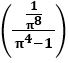A visual explanation: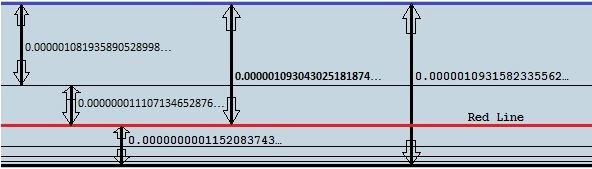(Perhaps these lines represent spectral lines)

0.020531964509368670378… / 18977.06203214114801425… = 0.0000010819358905289
0.020531964509368670378… / 18784.22352674753383243… = 0.0000010930430251818
0.020531964509368670378… / 18782.24385007314313978… = 0.0000010931582335562…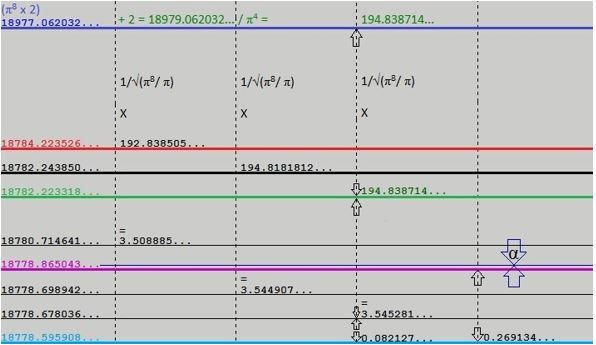18977.06203214114801425 - 192.83850539361418182 =
18784.22352674753383243 - 001.97967667439069265… =
18782.24385007314313978… - 000.02053196450936867… =
18782.22331810863377111

Dividing by π4:

192.83850539361418182… / 97.409091034002437236 =
...1.97967667439069265… / 97.409091034002437236 =
Etc.
...0.02053196450936867…

194.8387140325142431432… (π4/.5) + (2/π4)

Next step acquiring the end (bottom) number:

194.8387140325142431432/ √(π8/π) =
3.545281301022101405403… +
0.082127858037474681513… (8/π4) =
3.627409159059576086916…

18782.22331810863377 - 3.627409159059576086916… = 18778.59590894957419502…

18977.06203214114801425… Start # -
18778.59590894957419502 End # =
198.4661231915738192301… -
0.269134858448657890432… (explanation follows) = 198.1969883331251613…

18977.06203214114801425 - 198.1969883331251613… = 18778.86504380802285291
or
18778.59590894957419502+ 0.269134858448657890… = 18778.86504380802285291

While working on possibilities a major clue presented itself:
When I subtracted the following two numbers,

18778.865043837324229… original number from the top of the page -
18778.59590894957419502… end # =
0.26913488775003398… -
0.020531964509368670378… (2/π4) =
0.248602923240665309622… 1/x =
4.022478846847383539716…2 =
16.18033607333465644263…

and noticing phi numbers, I reasonably deduced that phi is part of the solution!
I decided to run with it and reverse the process starting with (√1.25 + .5) x 10 =

16.18033988749894848204… √ =
4.022479320953551960229… 1/x =
0.248602893939289220053… +
0.020531964509368670378… (2/π4) =
0.269134858448657890432+
18778.59590894957419502=
18778.86504380802285291… (original number now adjusted, and locked in by ɸ and π) =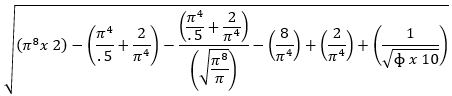137.035999079833116867867985771960524751261126710956764394872128728819663336
1/x
0.0072973525695056931682062262973500731680835106878962634963700448348384385

Phi is not included in the next and final section (see below), which is entirely pi based.

I do not believe that these two special numbers 3168 and 31680 are random numbers, especially
so close together, but were placed deliberately. If so, perhaps all those numbers are a language!
Wouldn't it be amazing if it says, "In the beginning God created the heaven and the earth." ?

Three interesting facts:

1. The value for the name “Lord Jesus Christ” in Greek numeric is 3168, the number of his name.

2. Earths dimensions fitted into a square has a perimeter of 31680 miles (7920 x 4).

3. He was born southeast of Bethlehem, at latitude 31.68 degrees North.

Inserting the final section to complete the formula: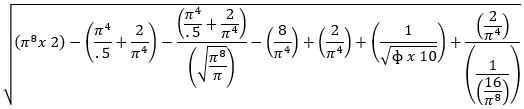137.035999206157399222318479001000262689537834609473995213691525285349733120
1/x
0.00729735256277875402980213640259738753519520968347474978298633008561682393

Observe the interesting number pattern in that last formula section: 1, 2, 4, 8, 16The first 3 sections span the distance between (π8 x 2) and the bottom line or end number.
From there the last 3 sections bounce back up to the alpha line, like a fold as in a curtain!

Speed of light in miles per second:

18778.8650438 -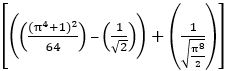= 18628.2396760… x 10 = 186282.396760…

18778.8650784-= 18628.2397106… x 10 = 186282.397106…